## Introduction :

In our dailylife, we observe different types of mirror. Different mirrors produce different images in different situation. Actually, mirror is the best example of smooth surface. Let’s discuss the different type of mirror.

## Types of Mirror :

Generally, there are three types of mirror.

1 ) Plane mirror

3 ) Parabolic mirror.

Let’s discuss each of the above mirror except parabolic mirror as it is included in syllabus of class 10 physics.

Firstly, we will discuss about the spherical mirror. We will learn about the Plane mirror in detail later.

## Spherical Mirror :

Spherical mirror is nothing but the mirror which is looked like a part of sphere. This mirror also has two types.

#### 1 ) Concave mirror :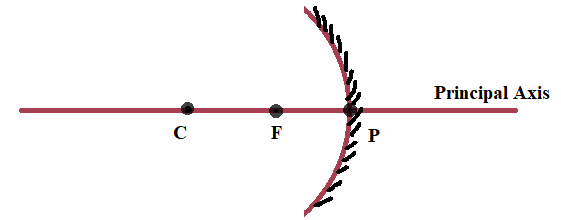The mirror silvered on the bulging side, is termed as the concave mirror.

#### 2 ) Convex mirror :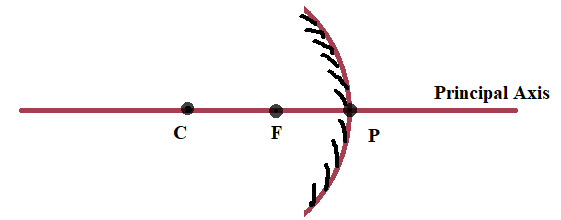The mirror silvered on the cave side is called as the convex mirror.

## Terms Related to Spherical Mirror :

### 1 ) Center of Curvature ( C ) :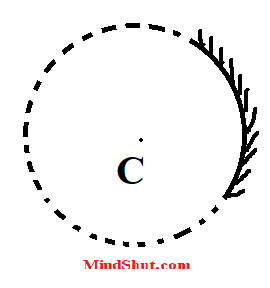Concave Mirror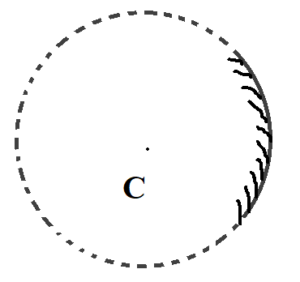Convex Mirror

A spherical mirror is looked like a part of a sphere. The center of that sphere is called as the center of curvature.

In the above figures, C is the center of curvature.

### 2 ) Principal Axis :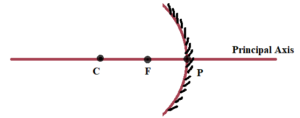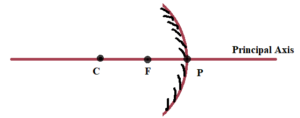The imaginary straight line passing through the center of curvature and aperture of a spherical mirror, is called as the Principal Axis.

In the above figures, red line indicates the principal axis.

### 3 ) Pole :The point of intersection of the aperture of the mirror and the principal axis is called as the pole of the mirror.

In the above figures, P is the pole of the spherical mirror.

### 4 ) Focus :When the rays of light parallel to the principal axis incident on the reflecting surface of a spherical mirror, after reflection, these rays converge at a single point or seemed to be diverged from a point. That point is called as Focus of the mirror. It lies on the principal axis. It is the midpoint of the distance between the center of curvature and pole of the mirror.

In the above figures, F is the focus of the spherical mirror.

### 5 ) Radius of curvature :The distance between the center of curvature and pole of the mirror, is called as the radius of the curvature.

In the above figures CP is radius of curvature.

### 6 ) Focal Length :The distance between the focus and pole of the spherical mirror is called as thse focal length. It is half of radius of curvature.

In the above figures, FP is the focal length.

### 7 ) Focusing Action of the Spherical Mirror :

When the rays of light parallel to the principal axis incident on the reflecting surface of a spherical mirror, after reflection, these rays converge at a single point or seemed to be diverged from a point. This point is nothing but the focus and this process or action of the mirror is termed as the focusing action of the spherical mirror.

## Rules for drawing the image formed by a spherical Mirror : ( For concave mirror )

1 ) When the rays of light parallel to the principal axis incident on the concave mirror, after reflection, the reflected rays pass through the focus.

2 ) When the incident ray of light passes through the focus, after reflection it would be parallel to the principal axis.

3 ) When the incident ray of light passes through or appeared to be passed through the center of curvature, after reflection, it would be reflected back in its own path.

4 ) When the rays of light incident on the concave mirror, it reflects according to the laws of reflection of light ( that is angle of incidence is equal to the angle of reflection )

## Rules for drawing the image formed by a spherical Mirror : ( For convex mirror )

1 ) When the rays of light parallel to the principal axis incident on the convex mirror, after reflection, the reflected rays appeared to be passed through the focus.

2 ) When the incident ray of light is appeared t be passed through the focus, after reflection it would be parallel to the principal axis.

3 ) When the incident ray of light passes through or appeared to be passed through the center of curvature, after reflection, it would be reflected back in its own path.

4 ) When the rays of light incident on the convex mirror, it reflects according to the laws of reflection of light ( that is angle of incidence is equal to the angle of reflection )

## Image formation By concave mirror :

1 ) Properties of the image produced by the concave mirror when the object is placed at infinity :

* The image is produced at focus of the mirror.

* The image is highly diminished or point size.

* The image is inverted.

* The image is real.

2) Properties of the image produced by the concave mirror when the object is placed beyond Center of curvature :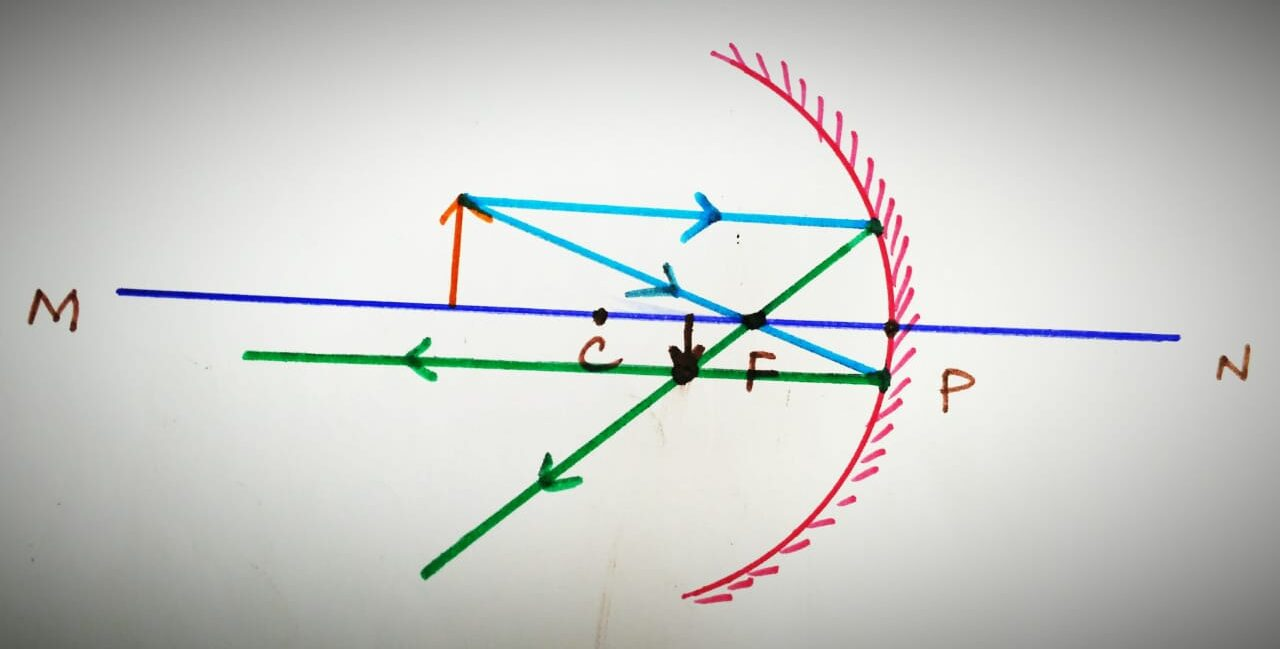* The image is produced between Center of curvature and focus.

* The image is diminished in size.

* The image is inverted.

* The image is real.

3 ) Properties of the image produced by the concave mirror when the object is placed at Center of curvature :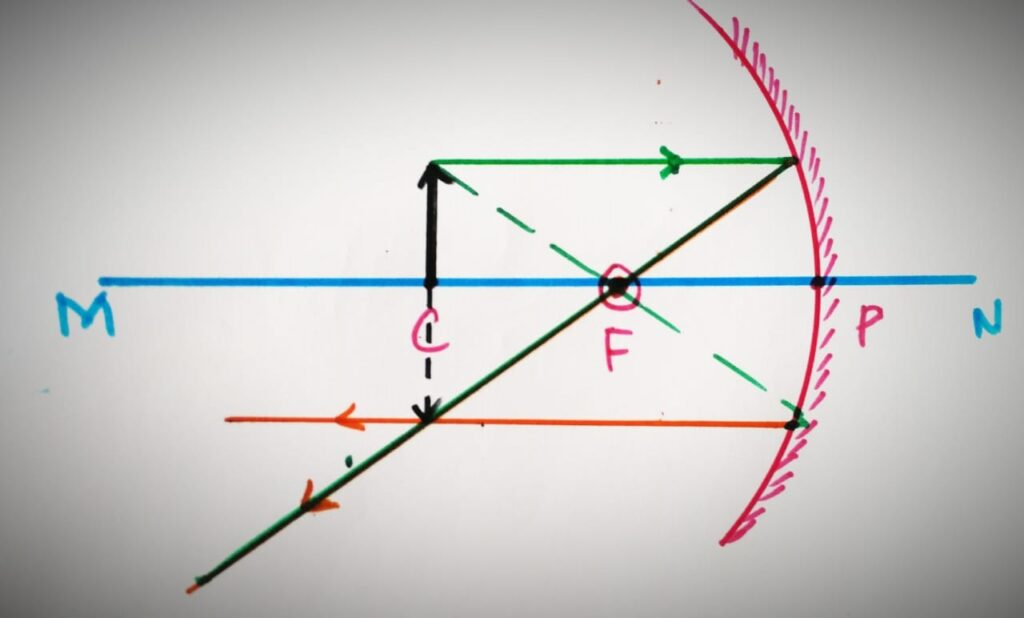* The image is produced at Center of curvature.

* The image is equal in size.

* The image is inverted.

* The image is real.

4 ) Properties of the image produced by the concave mirror when the object is placed between Center of curvature and focus :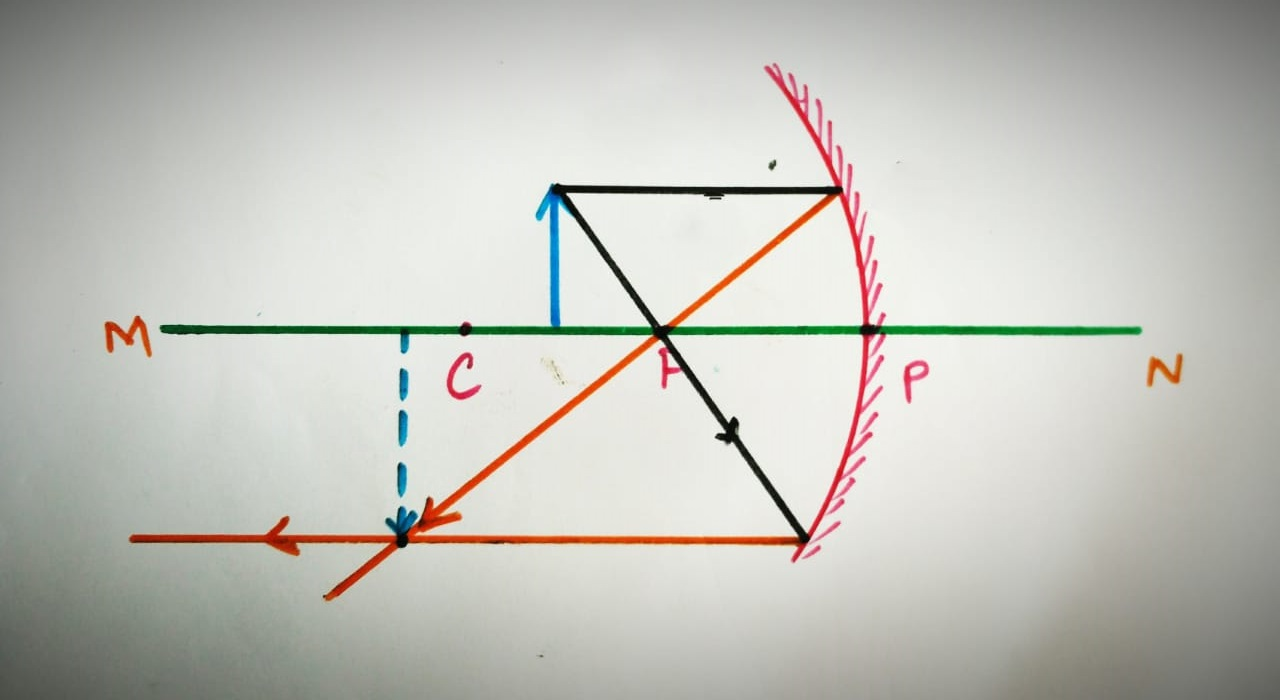* The image is produced beyond center of curvature .

* The image is enlarged in size.

* The image is inverted.

* The image is real.

5 ) Properties of the image produced by the concave mirror when the object is placed at Focus :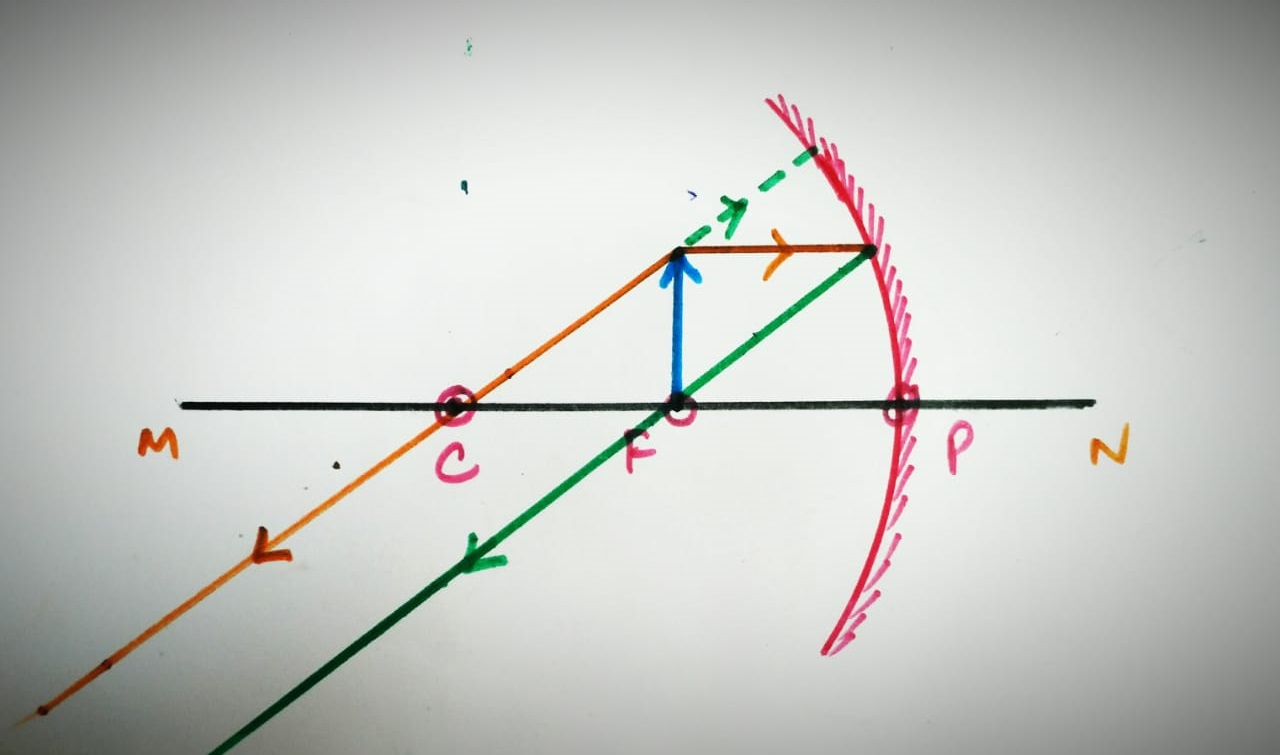* The image is produced at infinity.

* The image is highly magnified in size.

* The image is inverted.

* The image is real.

6 ) Properties of the image produced by the concave mirror when the object is placed between Focus and pole of the mirrror :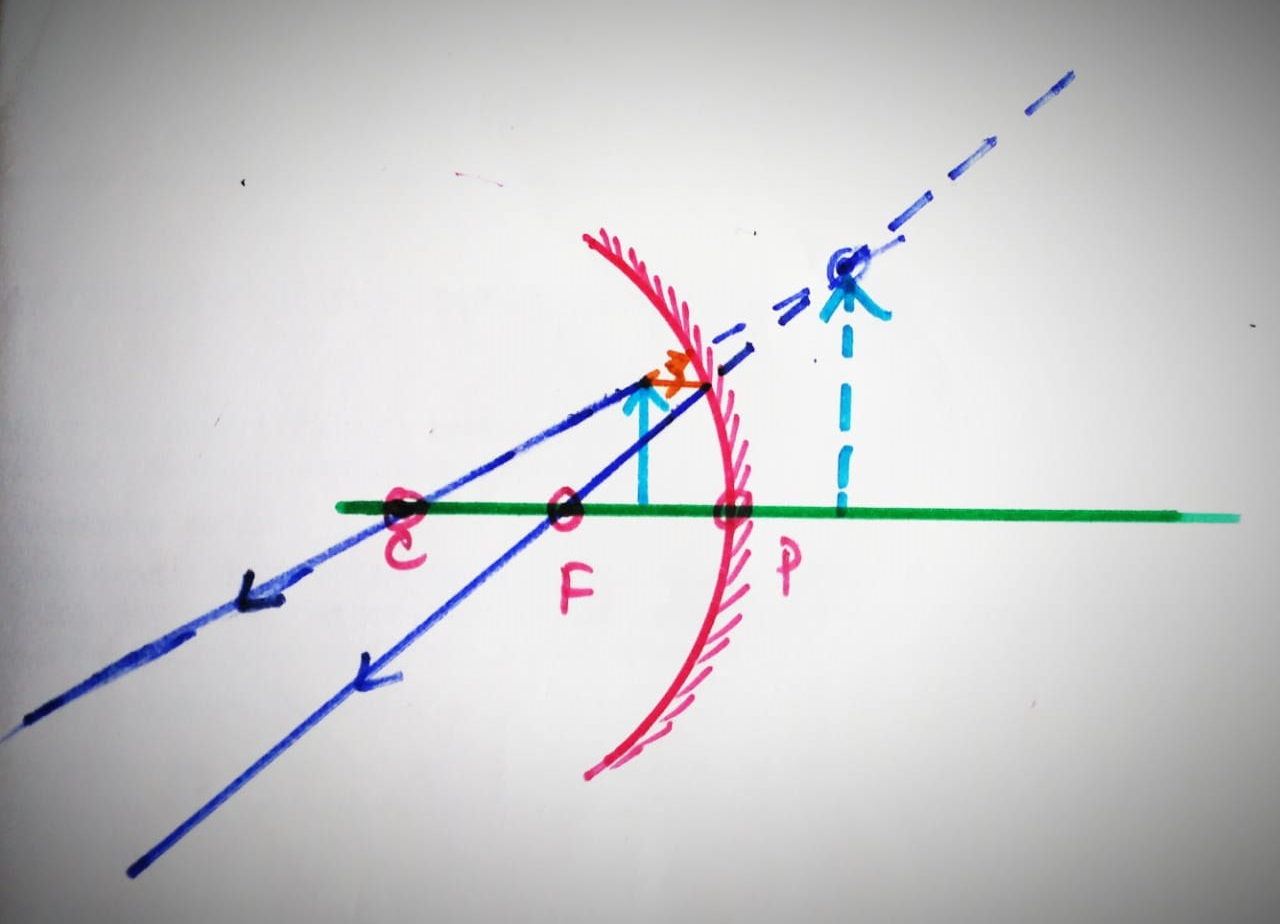* The image is produced behind the mirror.

* The image is enlarged in size.

* The image is erect.

* The image is virutual.

Conclusion :

1 ) When the object is moved towards the concave mirror, the size of the image increases and vise versa.

2 ) When the object is moved towards the concave mirror, the image distance from the mirror also increases except one situation in which the object is placed between the focus and pole of the mirror.